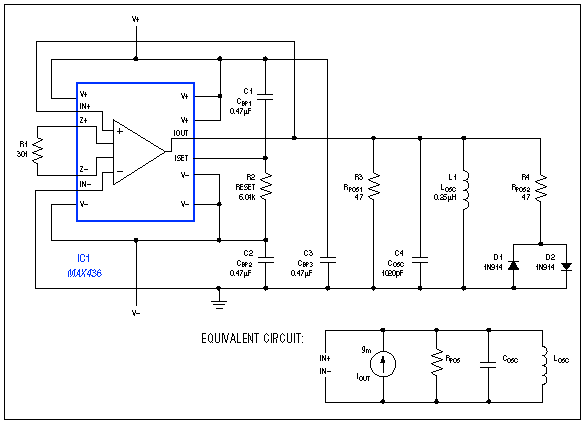# LC Oscillator Has 1% THD

### Abstract

At the heart of many oscillators is a parallel-resonant LC tank circuit whose impedance is infinite at the resonant frequency of 1/(2LC)Hz. Infinite impedance implies an absence of parallel damping resistance, so once it starts, an ideal tank circuit should continue oscillating indefinitely. The actual tank circuit, of course, has parasitic resistances that dissipate energy, causing the oscillations to die out. You can counteract this effect by adding a \"negative\" resistance, which cancels the net parallel parasitic resistance. Negative resistance is easily synthesized with a wideband transconductance amplifier (WTA). This 9.3MHz oscillator circuit includes a wideband transconductance amplifier, whose negative resistance counters losses in the LC tank circuit.

At the heart of many oscillators is a parallel-resonant LC tank circuit whose impedance is infinite at the resonant frequency of 1/(2LC)Hz. Infinite impedance implies an absence of parallel damping resistance, so once it starts, an ideal tank circuit should continue oscillating indefinitely.

The actual tank circuit, of course, has parasitic resistances that dissipate energy, causing the oscillations to die out. You can counteract this effect by adding a "negative" resistance, which cancels the net parallel parasitic resistance. Negative resistance is easily synthesized with a wideband transconductance amplifier (WTA).Figure 1. This 9.3MHz oscillator includes a wideband transconductance amplifier (IC1), whose negative resistance counters losses in the L1/C4 tank circuit.

Connect the WTA's positive input to its output and its negative input to ground (Figure 1). Then, a positive voltage applied to the output causes current to flow out of the amplifier, in proportion to the applied voltage. The circuit acts like a resistor whose current flows in the opposite direction; hence the negative value. (Note the equivalent circuit in Figure 1.)

The source impedance of IC1's current-source output (2.5kW minimum) is compatible with the 50W -to-300W load resistance in applications for which the IC is intended. The load resistance in this circuit (R3) also resembles that in a typical application. R3 should be much smaller than the tank-circuit parasitics, yet larger (in absolute value) than the WTA's negative resistance. R1 sets the negative resistance in terms of the amplifier's transconductance: gm = 8/R1, where the factor of eight is inherent in the IC.

The negative resistance value is therefore (R1)/8, which must be less than R3: (R1)/8 < R3. Choosing 47W for R3 yields R1 < 8R3 = 376W . A reasonable value for R1, therefore, is 301W . As intended, the parallel combination of negative resistance (-(R1)/8 = -37.6W ) and positive R3 (47W ) yields a negative resistance (-189W ) that shifts the oscillator's complex-conjugate pole pair to the right half plane.

By itself, the combination of tank circuit and regenerative element (negative resistance) simply drives the output amplitude to saturation. To achieve steady oscillation the circuit needs an amplitude limiter. R4 serves that purpose; it appears (in parallel with R3) only when the amplitude is sufficient to turn on one of the diodes D1 or D2.